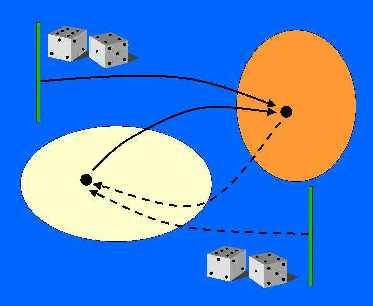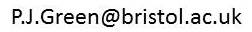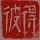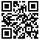Mathematics home Statistics home Postgrad opportunities Statistics clinicPeter Green Research Papers Collaborators Software Books Links Personal Statistical Science Editorial policy Future papers Instructions for authors SuSTaIn ACEMS Past Earth Network Royal Statistical Society International Society for Bayesian Analysis Royal Society

PJG Home / Software

### AutoRJ

-- a Fortran program for Unix-like systems, implementing the methodology for automatic reversible jump MCMC sampling described in section 6 (pages 191-4) of Green (Highly Structured Stochastic Systems, chapter 6, pages 179-206, OUP, 2003).
A new approach to automated reversible jump sampling has been taken in David Hastie's AutoMix sampler, which you may want to try instead of AutoRJ.If you download the files, please send a message to me at, with your email address and any comments. I can then keep you informed of any updates.
Update history
16 June 2003 - first posted
20 June 2003 - these instructions and readme.txt clarified
15 March 2005 - correction of (probably benign) error in AutoRJ.f, and expansion of all tabs in source files to spaces.
12 September 2012 - amended versions of AutoRJ.f and Makefile so that (a) source file can be compiled OK on compilers that impose traditional 72-character limit on source lines, (b) Makefile agrees with the one used in the example below

INSTALLATION

The package distributed consists of:
main source file        AutoRJ.f
auxiliary routines in
fortran and C     algama.f gauss4.f rgamma.f sd.c sokal.f
makefile                Makefile
example model files     toy.f dfnlp.f cptlp.f

It is provided as both a gzipped tarfile and as a zip archive.
In the former, the files all have Unix-style newline characters,
and in the latter Dos-style line endings. The makefiles should be
appropriate for Unix and Dos respectively.

For a free Unix-like shell that runs under Windows, try Cygwin
(www.cygwin.com).

The program has been run successfully:
using f77 and cc under Solaris on Sun workstations
using GNU compilers under Linux on Intel processors
using GNU compilers and Cygwin under Windows on Intel PCs

---------------------------------------------

USING AutoRJ

AutoRJ is a Fortran program for Unix-like systems, implementing
the methodology for automatic reversible jump MCMC sampling
described in section 6 (pages 191-4) of Green (Highly Structured
Stochastic Systems, chapter 6, pages 179-206, OUP, 2003).
These notes assume familiarity with this chapter.

In particular, possible users should carefully note the
warnings in that chapter about the lack of sophistication
of the approach to reversible jump embodied in this code.
The reliability of results from this sampler depends on
many factors, including the initial values and spread
parameters provided by the user, and the degree of multimodality
of the parameter (posterior) distribution within each
submodel.

The program is compiled with a user-provided Fortran or C
function that defines the model in question; when run, it
produces multiple output text files summarising the
MCMC sample, for subsequent analysis
and display (R or Splus are good choices for these
tasks). Only very limited summary information
is computed by this program itself.

---------------------------------------------

WRITING the Model Function

The model function has the structure, in Fortran:

real function target(k,params)
integer k
real params(*)

The function must be called 'target'.

Both arguments k and params must be variables:
k is an input parameter, which also controls the
k is read-only unless it takes the value 0 or
a value between kmax+1 and 2*kmax on input;
params is a real array, used for output for
certain values of k, and input for others.
The function has 5 distinct functions:

1. if k=0 on input, the function returns with k set to kmax,
the number of sub-models being entertained (which are indexed
1,2,..., kmax).

2. if k lies between kmax+1 and 2*kmax on input, it is
replaced by nk(k-kmax) on exit, where nk(j) is the
dimension n_j of submodel j.

3. if k lies between 2*kmax+1 and 3*kmax, then on exit,
params(j), j=1,2,...,nk(k-2*kmax) contains the initial
values for the parameters in the corresponding
submodel.

4. if k lies between 3*kmax+1 and 4*kmax, then on exit,
parameters for the parameters in the corresponding
submodel.

5. if k lies between 1 and kmax, then the function evaluates
the logarithm of the target distribution, up to an additive
constant, where k is given in the first argument k, and
the vector \theta_k in the second argument params(), in
consecutive entries starting at 1. The value of the
log-target is returned through the value of the
function target.

In a Bayesian model determination problem, the log-target
is the log-posterior, \log p(k,\theta_k|Y) in the notation
of the HSSS book chapter. Typically, one provides
simply \log p(k) + \log p(\theta_k|k) + \log p(Y|k,\theta_k),
the sum of log model prior probability, log parameter
prior density and log likelihood. By Bayes' theorem,
this is equivalent.

AutoRJ calls target once with k=0, and once for
each value of k from 1 to kmax, for functions 2, 3 and 4
above. Of course, it calls target to perform function 5
many times in the simulation.

Three examples are provided in the distribution - for the
two examples on page 193 of the HSSS book chapter, and
a simpler 'toy' example, a mixture of a univariate and
a bivariate density.

---------------------------------------------

COMPILING AutoRJ

Edit the file Makefile to specify the name of the
file containing model function (without the .f or .c
extension) as variable TARGET. Then type 'make'.
The compiled version will be named AutoRJ or
AutoRJ.exe as appropriate.

---------------------------------------------

RUNNING AutoRJ

The run of the program is controlled by options, switches
and parameter settings that have sensible default values,
and can be set by command line arguments in Unix shell
style.

The options are as follows. In this list, N denotes
a numerical value passed in as a parameter of the
sampler, other options are logical settings
that are by default off, turned on by specifying the
option.

Options

-nN         run sampler for N sweeps in within-model RWM
phase, and 10*N sweeps in model-jumping phase
-tN         use t distributions with N degrees of freedom
instead of normal distributions for proposing
innovation variables u
-p          switch on random permutation option (matrix R)
-seedN      random number seed (default 0, meaning use clock
time to initialise). In the log file for the run,
the seed actually used is printed, and the run
can be repeated with the same random numbers
by specifying that value in this option on the
subsequent run.
-save       save state (mu, B etc) after within-model RWM
phase, so that subsequent runs can omit this
phase and proceed directly to model jumping
-sN         thinning parameter for Sokal's estimate of
autocorrelation time: default N=1, no thinning
(N=0: suppress this computation)

The only other command-line argument supported (and which is
mandatory) is the name of a subdirectory of the current directory,
which is used for all the output files created by the run, and
for the save-state information, if the -save option is used.

All the output files are given names of the
form N_ext, where N is a unique integer assigned by the program
to label successive runs (it chooses the smallest positive integer
such that N_log does not already exist), and _ext signifies the
type of output the file contains:

_log        records information about the run
_model      time-series of the model indicator k, thinned if
the run is very long (to give a maximum of 10000 values)
_parK       for K=1,2,..., time-series of the sampled
model-K parameters, thinned if the run is very long
(to give a maximum of 5000 parameter sets over
all sub-models)
_acf        autocorrelation function for the model indicator

---------------------------------------------

EXAMPLE of compiling and running AutoRJ

(a)

~/AutoRJDist  cat Makefile
FC = g77
CC = gcc
FFLAGS = -O2
TARGET = dfnlp

AutoRJ.exe: AutoRJ.o $(TARGET).o sd.o gauss4.o rgamma.o algama.o sokal.o$(FC) $(FFLAGS) -o AutoRJ.exe AutoRJ.o$(TARGET).o \
sd.o gauss4.o rgamma.o algama.o sokal.o
sd.o: sd.c
$(CC) -c -o sd.o -DRETS -DSUNF sd.c ~/AutoRJDist$ $make g77 -O2 -c -o AutoRJ.o AutoRJ.f g77 -O2 -c -o dfnlp.o dfnlp.f gcc -c -o sd.o -DRETS -DSUNF sd.c g77 -O2 -c -o gauss4.o gauss4.f g77 -O2 -c -o rgamma.o rgamma.f g77 -O2 -c -o algama.o algama.f g77 -O2 -c -o sokal.o sokal.f g77 -O2 -o AutoRJ.exe AutoRJ.o dfnlp.o \ sd.o gauss4.o rgamma.o algama.o sokal.o (b) ~/AutoRJDist$ mkdir dfn

(c)

~/AutoRJDist $AutoRJ dfn -n10000 auto-rj nsweep = 10000 seed = 1055525484 normal proposals run: dfn/1 ... setting up, doing rwm for k = 1( 70) 2( 57) 3( 55) 4( 48) 5( 39) kmax = 5 nk: 1 2 2 3 4 ... auto-jumping: 9 8 7 6 5 4 3 2 1 0 model frequencies: 507 49152 1064 44117 5160 percentage jumps accepted: 29.4 nkeep, nsokal, tau 32768 1 2.8256 (d) ~/AutoRJDist$ ls dfn
1_acf  1_par1  1_par2  1_par3  1_par4  1_par5  1_log  1_model

This shows

(a) compilation
(b) creation of subdirectory for output files
(c) run of AutoRJ, with output showing:
number of sweeps
random number seed to repeat
type of proposal distribution
index number of run
RWM phase, with percentage acceptances in each submodel
number of submodels
dimensions of submodels
countdown of main model-jumping run
model frequencies
percentage acceptance of model-jumping moves
autocorrelation time statistics.
(d) list of output files in subdirectory



AutoRJ is free of charge for educational and non-commercial research purposes.
Please report any problems with the code to the author, Peter Green, via Email link

 Professor Peter Green, School of Mathematics, University of Bristol, Bristol, BS8 1TW, UK. Email link Telephone: +44 (0)117 928 7967; Fax: +44 (0)117 928 7999